Arrays
Number Bonds
Tape Diagrams
Equal Groups
Vocabulary
100

What multiplication sentence represents this array?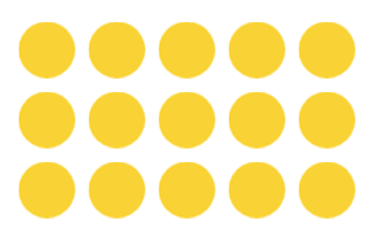3 x 5 = 15

100

What is the missing multiplication equation that completes the number bond?( 2 x 5 )

100

What multiplication sentence represents this tape diagram?4 x 3 = 12

100

What multiplication sentence represents these groups?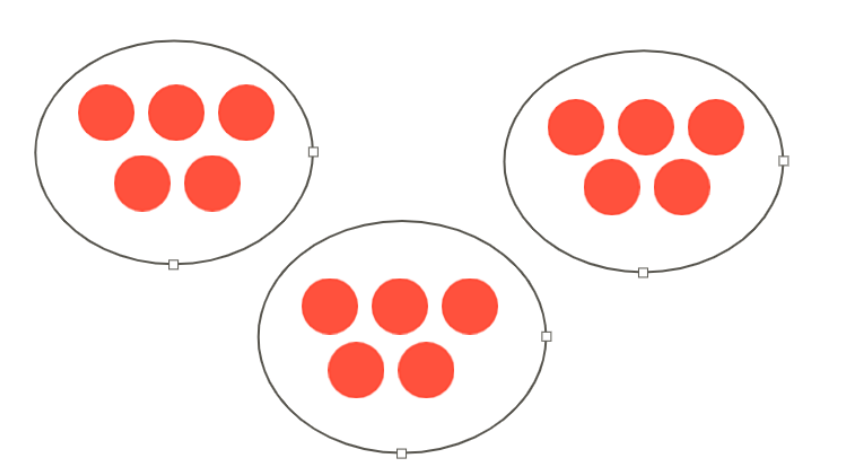3 x 5 = 15

100

The answer to a multiplication problem.

Product

200

What division sentence represents the array?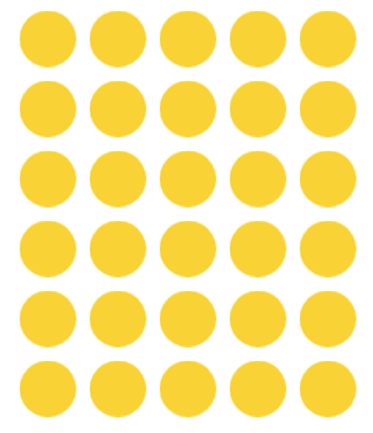30 -: 5 = 6

200

What is the missing multiplication equation that completes the number bond?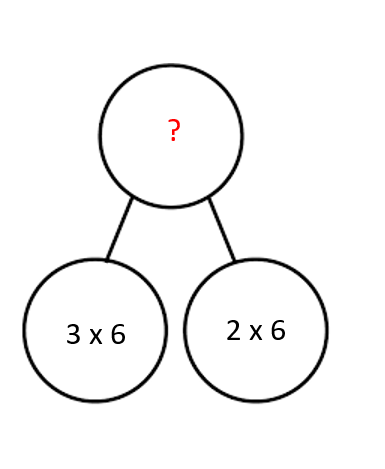(5 x 6)

200

What division sentence would represent this tape diagram?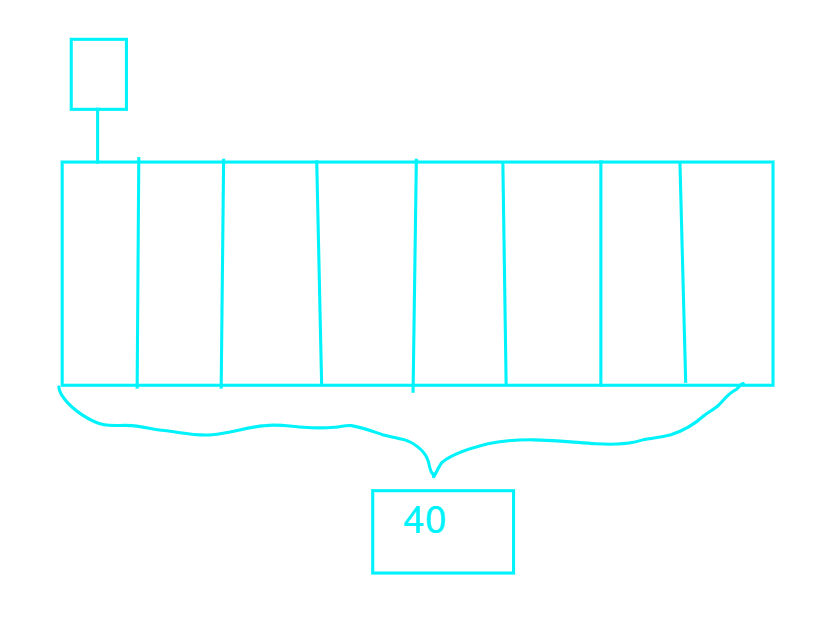40 -: 5 = 8

200

What division sentence represents these equal groups?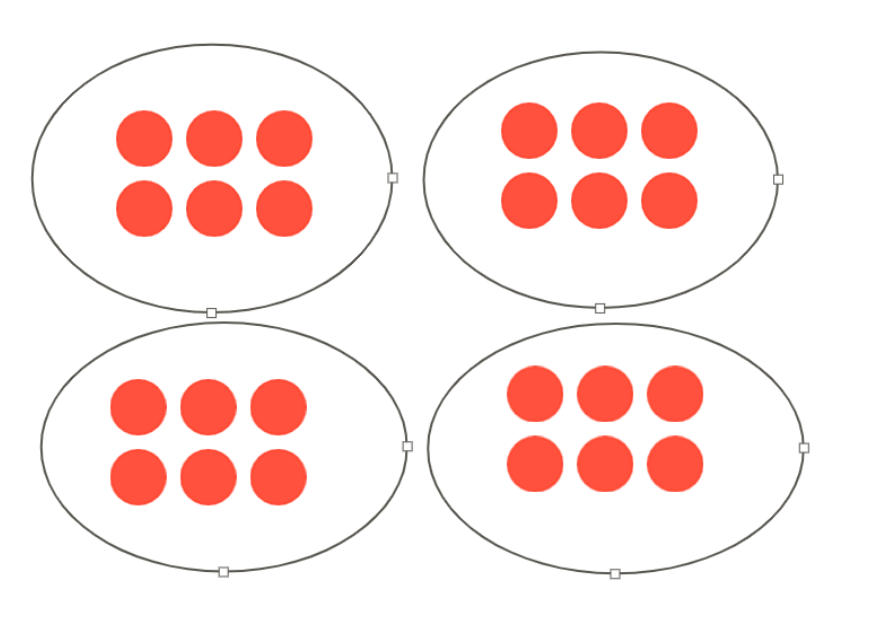24 -: 6 = 4

200

The special name for the numbers that are multiplied together in a multiplication sentence.

Factors

300

Using distributive property, how would you represent a multiplication sentence for this array?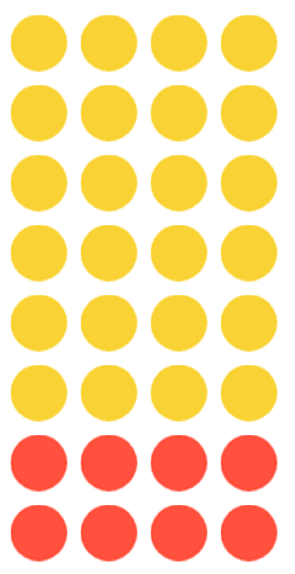(6 x 4) + (2 x 4) = 8 x 4

300

What is the complete multiplication equation that represents the number bond you see below?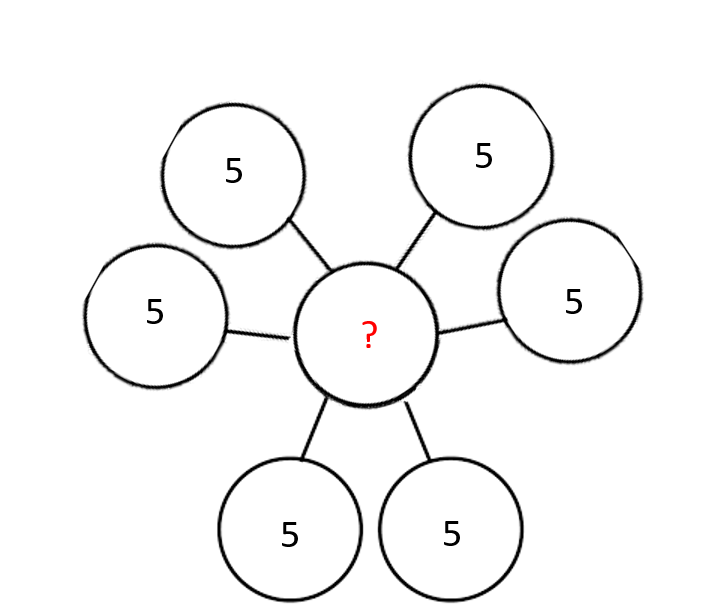6 x 5 = 30

300

Mr. Threeton divided up all the bags of candy between the 4 third grade classrooms.  How many bags of candy did 2 of the classrooms get?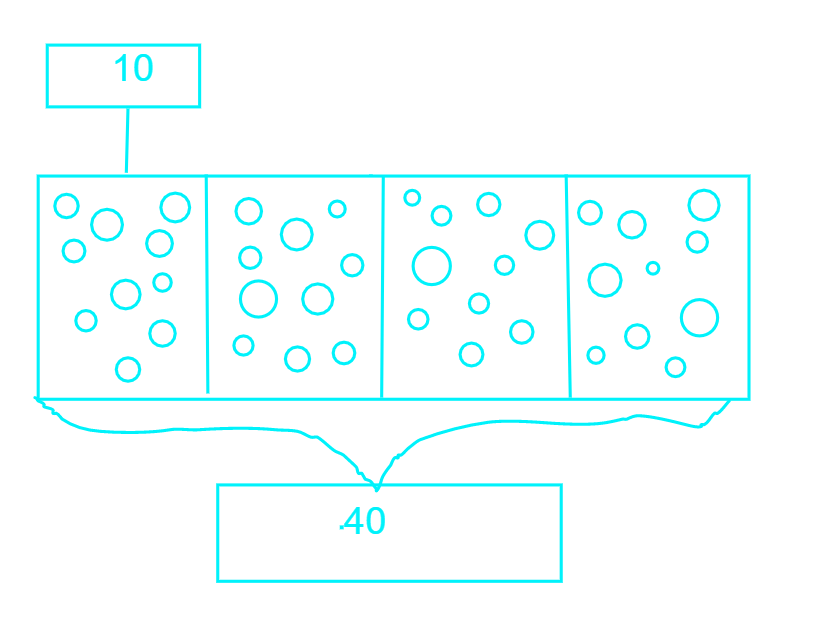20 bags of candy

300

What multiplication sentence best represents these equal groups?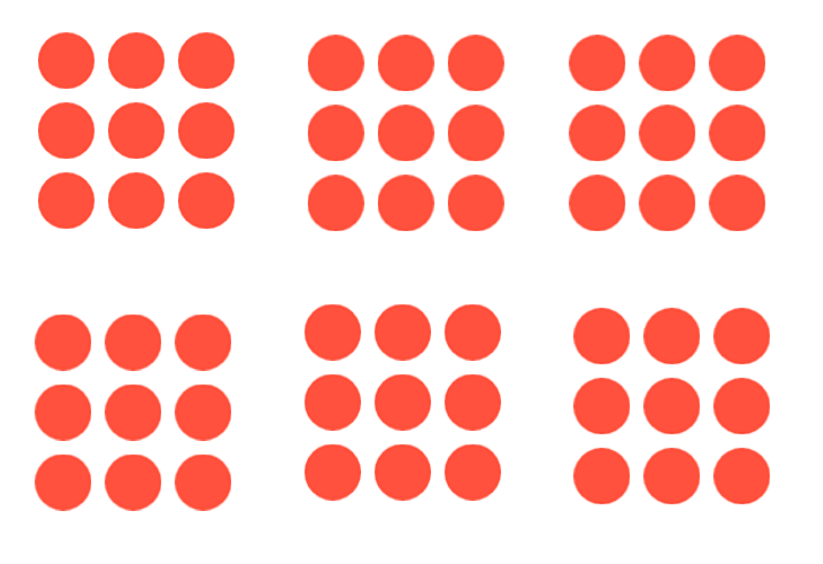6 x 9 = 54

300

The answer in a division problem.

Quotient

400

Using distributive property, show how you would represent this array using division.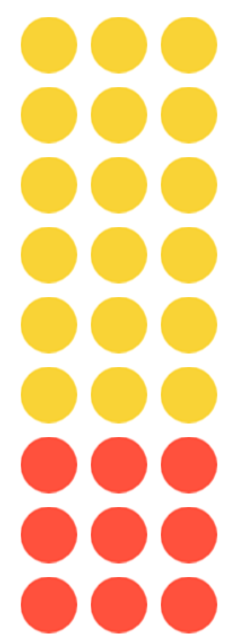(18 -: 3) + (9 -: 3) = 27 -: 3

400

What multiplication equation would represent this number bond?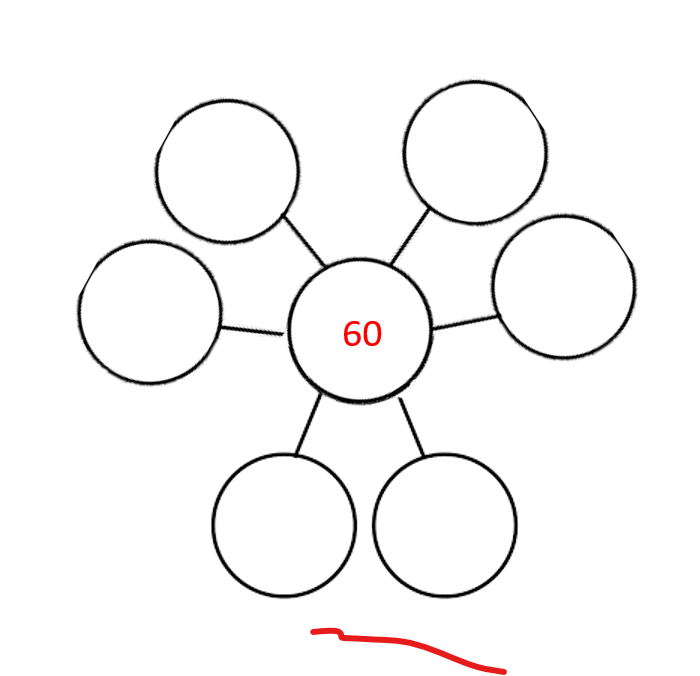6 x 10 = 60

400

There are 60 children to be divided equally into teams.  How many teams will there be?  How many children will there be on each team?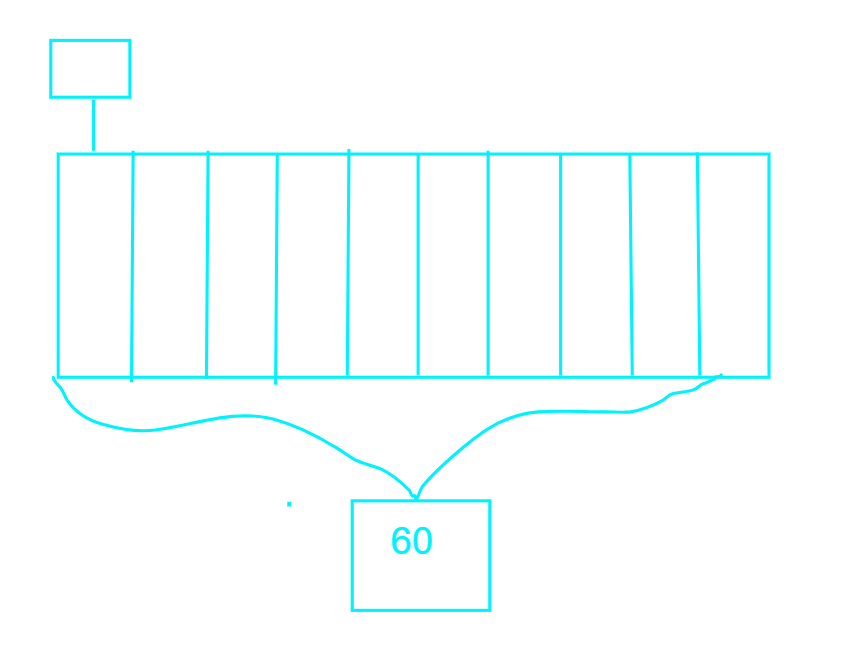There will be 10 teams.

There will be 6 children on each team.

400

What division sentence would represent these equal groups?54 -: 6 = 9

400

Name 3 ways to represent a multiplication or division equation with a drawing.

(Any 3) Draw equal groups,  a bar model or tape diagram, a number bond, an array, a number line

500

Draw an array to represent 15 divided by 3.

Teacher looks at student's array and awards points.

500

Look at the number bond on Jocelyn's screen.

Write a the FOUR equations that represent this number bond.

24 divided by 4= 6

24 divided by 6= 4

4 X 6= 24

6 x 4 = 24

500

Draw a bar model or tape diagram to represent this comparison problem.

Atticus' grandmother is 70 years old. She is 7 times older than Atticus. How old is Atticus?

Teacher will look at student's models and award points for correct models.

500

Draw an equal groups drawing for the problem below.

Jocelyn has 6 marbles in her collection. Caroline's collection is 3 times bigger. How many marbles are in Caroline's collection.

Teacher looks at student's drawing and awards points if it is correct.

500

How are area models and arrays similar?

How are they different?

Both have dimensions or lengths and widths.

Both make rectangles.

Both are used to model multiplication (and division)

Arrays show individual symbols (ex: ❤️⭐) in rows and columns.

Area models have square units organized in rows and columns, but you can't always see them.

Click to zoom